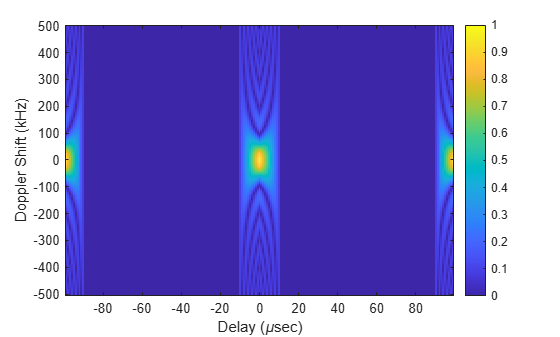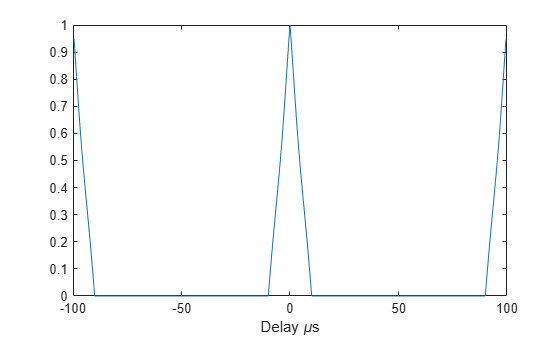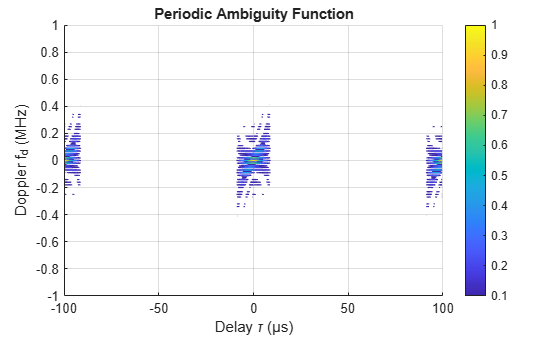# pambgfun

Periodic ambiguity function

## Syntax

pafmag = pambgfun(X,fs)
[pafmag,delay,doppler] = pambgfun(X,fs)
[pafmag,delay,doppler] = pambgfun(X,fs,P)
[pafmag,delay] = pambgfun(___,'Cut','Doppler')
[pafmag,delay] = pambgfun(___,'Cut','Doppler','CutValue',V)
[pafmag,doppler] = pambgfun(___,'Cut','Delay')
[pafmag,doppler] = pambgfun(___,'Cut','Delay','CutValue',V)
[pafmag,delay,doppler] = pambgfun(___,'Cut','2D')
pambgfun(___)

## Description

example

pafmag = pambgfun(X,fs) returns the magnitude of the normalized periodic ambiguity function (PAF) for one period of the periodic signal X. fs is the sampling rate.

example

[pafmag,delay,doppler] = pambgfun(X,fs) also returns the time delay vector, delay, and the Doppler shift vector, doppler. The delay vector is along the zero Doppler cut of the PAF. The Doppler shift vector is along the zero delay cut.

example

[pafmag,delay,doppler] = pambgfun(X,fs,P) returns the magnitude of the normalized PAF for P periods of the periodic signal X.

example

[pafmag,delay] = pambgfun(___,'Cut','Doppler') returns the PAF, pafmag, along a zero Doppler cut. The delay argument contains the time delay vector corresponding to the columns of pafmag.

example

[pafmag,delay] = pambgfun(___,'Cut','Doppler','CutValue',V) returns the PAF, pafmag, along a nonzero Doppler cut specified by V. The delay argument contains the time delay vector corresponding to the columns of pafmag.

example

[pafmag,doppler] = pambgfun(___,'Cut','Delay') returns the PAF, pafmag, along the zero delay cut. The doppler argument contains the Doppler shift vector corresponding to the rows of pafmag.

example

[pafmag,doppler] = pambgfun(___,'Cut','Delay','CutValue',V) returns the PAF, pafmag, along a nonzero delay cut specified by V. The doppler argument contains the Doppler shift vector corresponding to the rows of pafmag.

example

[pafmag,delay,doppler] = pambgfun(___,'Cut','2D') returns the PAF, pafmag, for all delays and Doppler shifts. The doppler argument contains the Doppler shift vector corresponding to the rows of pafmag. The delay argument contains the time delay vector corresponding to the columns of pafmag. You cannot use 'CutValue' when 'Cut' is set to '2D'.

example

pambgfun(___) with no output arguments plots the PAF. When 'Cut' is '2D', the function produces a contour plot of the PAF function. When 'Cut' is 'Delay' or 'Doppler', the function produces a line plot of the PAF cut.

## Examples

collapse all

Plot the PAF function of a rectangular pulse waveform for one period. Assume the pulse repetition frequency (PRF) is 10.0 kHz and that the sampling frequency is a multiple of the PRF.

PRF = 10.0e3; fs = 101*PRF; waveform = phased.RectangularWaveform('SampleRate',fs,'PulseWidth',1e-5, ... 'NumPulses',1,'PRF',PRF); wav = waveform(); pamf = pambgfun(wav,fs); imagesc(pamf) axis equal axis tightPlot the periodic ambiguity function of a rectangular pulse waveform for one period. Assume the pulse repetition frequency (PRF) is 10.0 kHz and that the sampling frequency is a multiple of the PRF. Return the Doppler and delay values from the pambgfun function.

PRF = 10.0e3; fs = 101*PRF; waveform = phased.RectangularWaveform('SampleRate',fs,'PulseWidth',1e-5, ... 'NumPulses',1,'PRF',PRF); wav = waveform(); [pamf,delays,doppler] = pambgfun(wav,fs);

Plot the periodic ambiguity function.

imagesc(delays*1e6,doppler/1000,pamf) axis xy xlabel('Delay (\musec)') ylabel('Doppler Shift (kHz)') colorbarPlot a cut at zero delay for the periodic ambiguity function of a rectangular pulse waveform for five periods. Assume the pulse repetition frequency is 10.0 kHz and that the sampling frequency is a multiple of the PRF. Return the Doppler and delay values from the function.

PRF = 10.0e3; fs = 101*PRF; waveform = phased.RectangularWaveform('SampleRate',fs,'PulseWidth',1e-5, ... 'NumPulses',1,'PRF',PRF); wav = waveform();

Find the periodic ambiguity functions along a zero delay cut for one and for five periods.

[pamf,delays,doppler] = pambgfun(wav,fs,1); f1 = pamf(:,101); [pamf,delays,doppler] = pambgfun(wav,fs,5); f2 = pamf(:,101);

Plot the periodic ambiguity functions.

plot(doppler/1000,f1) hold on plot(doppler/1000,f2) xlabel('Doppler Shift (kHz)') legend('One-Period PAF','Five-Period PAF')Plot the zero Doppler cut for the five-period periodic ambiguity function of a linear FM pulse waveform. Assume the pulse repetition frequency (PRF) is 10.0 kHz and that the sampling frequency is a multiple of the PRF. Return the Doppler and delay values from the pambgfun function.

PRF = 10.0e3; fs = 200*PRF; waveform = phased.LinearFMWaveform('SampleRate',fs,'PulseWidth',1e-5, ... 'NumPulses',1,'PRF',PRF); wav = waveform();

Find the five-period periodic ambiguity function along a zero Doppler cut.

[pamf,delays] = pambgfun(wav,fs,5,'Cut','Doppler');

Plot the periodic ambiguity functions.

plot(delays*1.0e6,pamf) xlabel('Delay \mus')Plot a non-zero Doppler cut for the 5-period periodic ambiguity function of a linear FM pulse waveform by explicitly specifying the cut value. Assume the pulse repetition frequency is 10.0e3 Hz and that the sampling frequency is a multiple of the PRF. Return the Doppler and delay values from the function.

PRF = 10.0e3; fs = 200*PRF; waveform = phased.LinearFMWaveform('SampleRate',fs,'PulseWidth',1e-5,... 'NumPulses',1,'PRF',PRF); wav = waveform();

Find the 5-period periodic ambiguity function along a non-zero Doppler cut.

dopval = 20.0; [pamf,delays] = pambgfun(wav,fs,5,'Cut','Doppler','CutValue',dopval);

Plot the periodic ambiguity functions.

plot(delays*1.0e6,pamf) xlabel('Delay \mus')Plot a zero delay cut for the three-period periodic ambiguity function of an FMCW waveform. Assume a sweep bandwidth of 100 kHz with a sampling frequency of 1 MHz. Return and plot the Doppler shift values.

fs = 1.0e6; waveform = phased.FMCWWaveform('SweepBandwidth',100.0e3,'SampleRate',fs, ... 'OutputFormat','Sweeps','NumSweeps',1); wav = waveform();

Find the three-period periodic ambiguity function along a zero delay cut.

[pamf,doppler] = pambgfun(wav,fs,3,'Cut','Delay');

Plot the zero delay cut of the periodic ambiguity function.

plot(doppler/1.0e3,pamf) xlabel('Doppler Shift (kHz)')Plot a non-zero delay cut of -20 μs for the three-period periodic ambiguity function of an FMCW waveform. Assume a sweep bandwidth of 100 kHz with a sampling frequency of 1 MHz. Return and plot the Doppler shift values.

fs = 1.0e6; waveform = phased.FMCWWaveform('SweepBandwidth',100.0e3,'SampleRate',fs, ... 'OutputFormat','Sweeps','NumSweeps',1,'SweepTime',100e-6); wav = waveform();

Find the three-period periodic ambiguity function along a nonzero Delay cut.

delayval = -20.0e-6; [pamf,doppler] = pambgfun(wav,fs,3,'Cut','Delay','CutValue',delayval);

Plot the nonzero delay cut of the periodic ambiguity function.

plot(doppler/1.0e3,pamf) grid xlabel('Doppler Shift (kHz)')Display an image of the 9-period periodic ambiguity function for a linear FM pulse waveform. Assume the pulse repetition frequency is 10.0e3 Hz and that the sampling frequency is a multiple of the PRF.

PRF = 10.0e3; fs = 200*PRF; waveform = phased.LinearFMWaveform('SampleRate',fs,'PulseWidth',1e-5,... 'NumPulses',1,'PRF',PRF); wav = waveform();

Compute and display the 9-period periodic ambiguity function for all delays and frequencies.

[pamf,delays,doppler] = pambgfun(wav,fs,9,'Cut','2D'); imagesc(delays*1e6,doppler/1e6,pamf) title('Periodic Ambiguity Function') xlabel('Delay \tau ({\mu}s)') ylabel('Doppler Shift (MHz)') axis xyPlot the seven-period periodic ambiguity function of a linear FM pulse waveform. Assume the pulse repetition frequency (PRF) is 10.0 kHz and that the sampling frequency is a multiple of the PRF.

PRF = 10.0e3; fs = 200*PRF; waveform = phased.LinearFMWaveform('SampleRate',fs,'PulseWidth',1e-5, ... 'NumPulses',1,'PRF',PRF); wav = waveform();

Find the periodic ambiguity function.

pambgfun(wav,fs,7,'Cut','2D')## Input Arguments

collapse all

Input pulse waveform, specified as a complex-valued vector.

Example: [0,.1,.3,.4,.3,.1.0]

Data Types: double
Complex Number Support: Yes

Sampling frequency, specified as a positive scalar. Units are in hertz.

Example: 3e3

Data Types: double

Number of periods, specified as a positive integer.

Example: 5

Data Types: double

When you set 'Cut' to 'Delay' or 'Doppler', use V to specify a nonzero cut value. You cannot use V when you set 'Cut' to '2D'.

When 'Cut' is set to 'Delay', V is the time delay at which the cut is taken. Time delay units are in seconds.

When 'Cut' is set to 'Doppler', V is the Doppler frequency shift at which the cut is taken. Doppler units are in hertz.

Example: 10.0

Data Types: double

## Output Arguments

collapse all

Normalized PAF function magnitude, returned as a vector or a matrix of nonnegative real values. The dimensions of pafmag depend on the value of 'Cut'.

'Cut'pafmagdimensions
'2D'M-by-N matrix.
'Delay'M-element column vector.
'Doppler'N-element row vector.

M is the number of Doppler frequencies and N is the number of time delays.

Time delay vector, returned as an N-by-1 vector. If N is the length of signal X, then the delay vector consist of 2N – 1 samples in the range, –(N/2) – 1,...,(N/2) – 1).

Doppler shift vector, returned as an M-by-1 vector of Doppler frequencies. The Doppler frequency vector consists of M = 2ceil(log2 N) equally-spaced samples. Frequencies are (–(M/2)Fs,...,(M/2–1)Fs).

collapse all

### Periodic Ambiguity Function

The periodic ambiguity function (PAF) is an extension of the ordinary ambiguity function to periodic waveforms.

Use this function analyze the response of a correlation receiver to a time-delayed or Doppler-shifted narrowband periodic waveform. Narrowband periodic signals consist of CW tones modulated by a periodic complex envelope. These types of signals are commonly used in radar systems to form transmitted pulse trains.

A time periodic waveform has the property y(t + T) = y(t), where T is the period. The PAF function for an N-period waveform is defined as

${A}_{NT}\left(\tau ,\nu \right)=\frac{1}{NT}{\int }_{0}^{NT}y\left(t+\frac{\tau }{2}\right){y}^{*}\left(t-\frac{\tau }{2}\right){e}^{i2\pi \nu t}dt$

Taking advantage of the periodicity, you can rewrite the function as

${A}_{NT}\left(\tau ,\nu \right)=\frac{1}{NT}\sum _{n=1}^{N}{e}^{i2\pi \nu \left(n-1\right)T}{\int }_{0}^{T}u\left(t+\frac{\tau }{2}\right){u}^{*}\left(t-\frac{\tau }{2}\right){e}^{i2\pi \nu s}ds$

The last term on the right side is the one-period PAF function, AT(τ,ν). The first term on the right side is due to Doppler only. The Doppler term is proportional to the periodic sinc() function and you can rewrite the periodic ambiguity function as

${A}_{NT}\left(\tau ,\nu \right)=\frac{\mathrm{sin}2\pi \nu NT}{N\mathrm{sin}2\pi \nu T}{e}^{i2\pi \nu \left(N-1\right)T}{A}_{T}\left(\tau ,\nu \right)$

The Doppler term improves the Doppler resolution by a factor of 1/NT.

The one-period PAF function is not the same as the ordinary ambiguity because the integration limits are different.

 Levanon, N. and E. Mozeson. Radar Signals. Hoboken, NJ: John Wiley & Sons, 2004.

 Mahafza, B. R., and A. Z. Elsherbeni. MATLAB® Simulations for Radar Systems Design. Boca Raton, FL: CRC Press, 2004.

 Richards, M. A. Fundamentals of Radar Signal Processing. New York: McGraw-Hill, 2005.